# Measurement Worksheets

#### What Are the Systems of Measurement?

Connecting different numbers or values with physical quantities and phenomena is the process that comes under the systems of measurements. Today, the concept of measures and weights includes different factors like an electric current, pressure, luminosity, temperature, etc. The only foundational measurement is an area, volume, mass, length, distance, mass, and weight. We measure liquid or grain in volume. The closely associated measurements of units are volume, length, and area. Standards, uniformity, and units are the concept that experts consider a foundational aspect of weights and measures. Suppose you need exact and reliable standard system of mass and length of different objects. In that case, we will use uniformity as it is the essence of systems of measurements. Units of measurements are the number of things like kilogram or pound, etc. According to the International Bureau of Weights and Measures, the standard kilogram is the standard of physical embodiment of a unit. Anyone can identify and assign a numerical value of any given quantity is the basic theory of measurement. The measurement system is always based on the nature of property measurement. There is a sign of objectivity in quantifying different things in their specific unit. It means we can represent similar quantities through different scales based on various factors.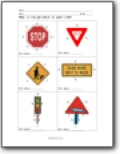###### Area and Perimeter

Students will learn how to find the area, circumference, and perimeter of various shapes.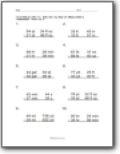###### Operations With Measurement

Students will learn how to process basic math operations with all different forms of measurement.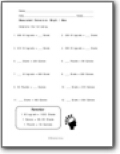###### Unit Conversion

Students will begin to understand how to process all types of different unit conversions between the U.S. standard form and metric system.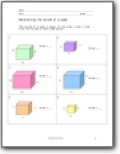###### Volume

Learn how to determine the amount of space that a geometric shape takes up.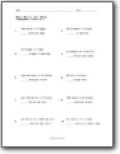###### Unit Rates

You will convert a math statement to a unit rate.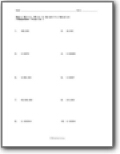###### Write in Scientific Notation

You are given a standard form value and asked to write it in scientific notation.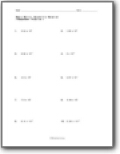###### Scientific Notation to Standard Form

You are given a value written in scientific notation. Convert that value to standard form.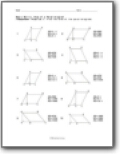###### Area of a Parallelogram

Find the area of the parallelograms.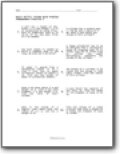###### Volume Word Problems

These word problems a ton of different shapes and measures. Here is an example: A large cylindrical can is to be designed from a rectangular piece of aluminum that is 25 inches long and 10 inches high by rolling the metal horizontally. Determine the volume of the cylinder.